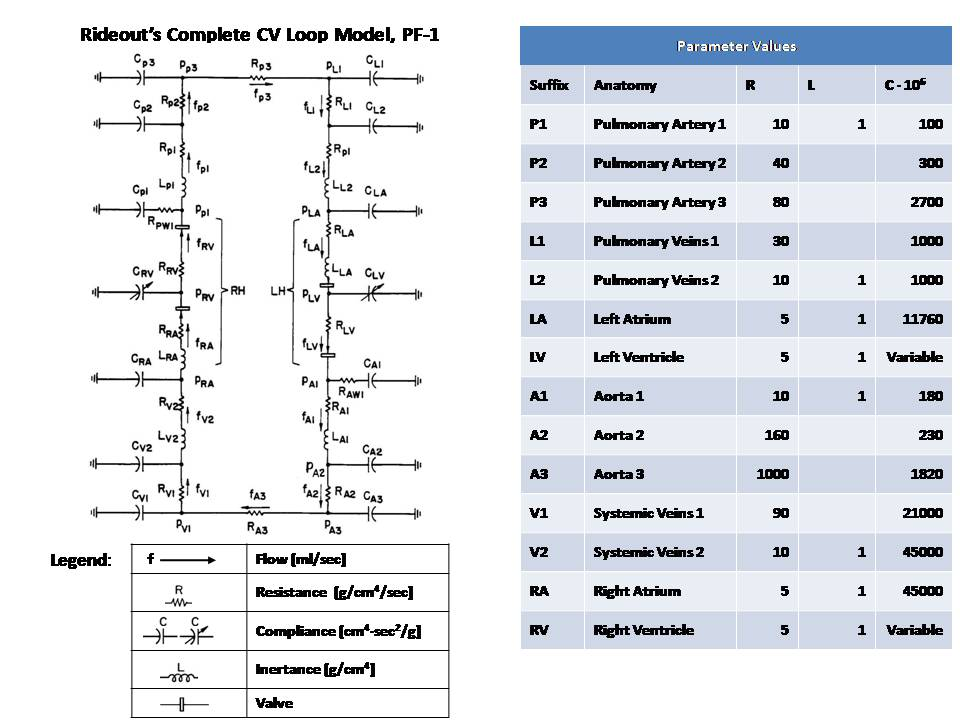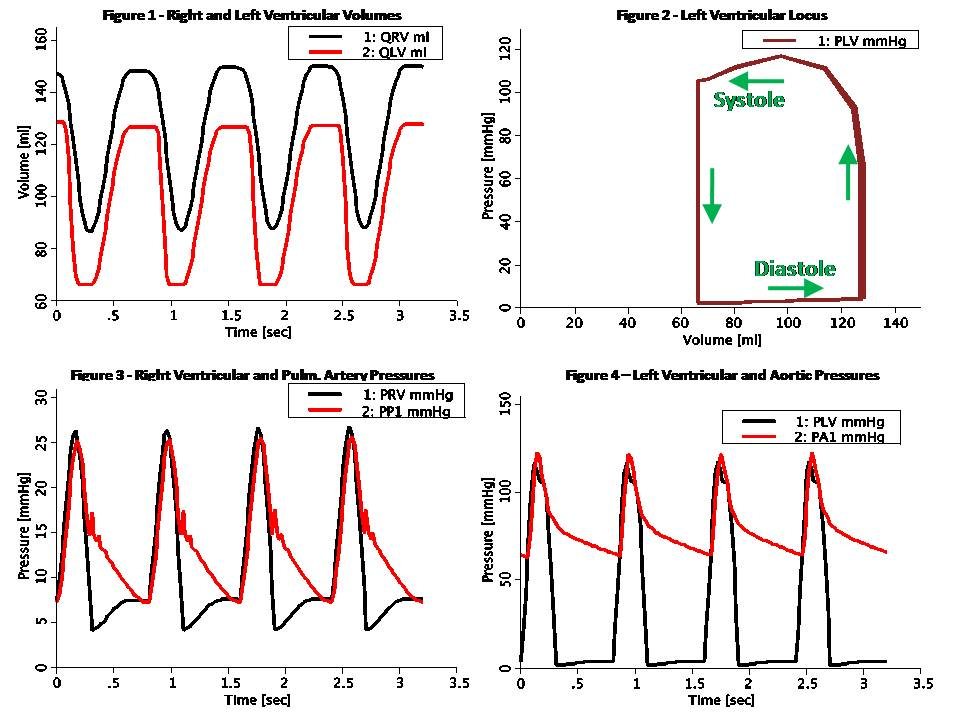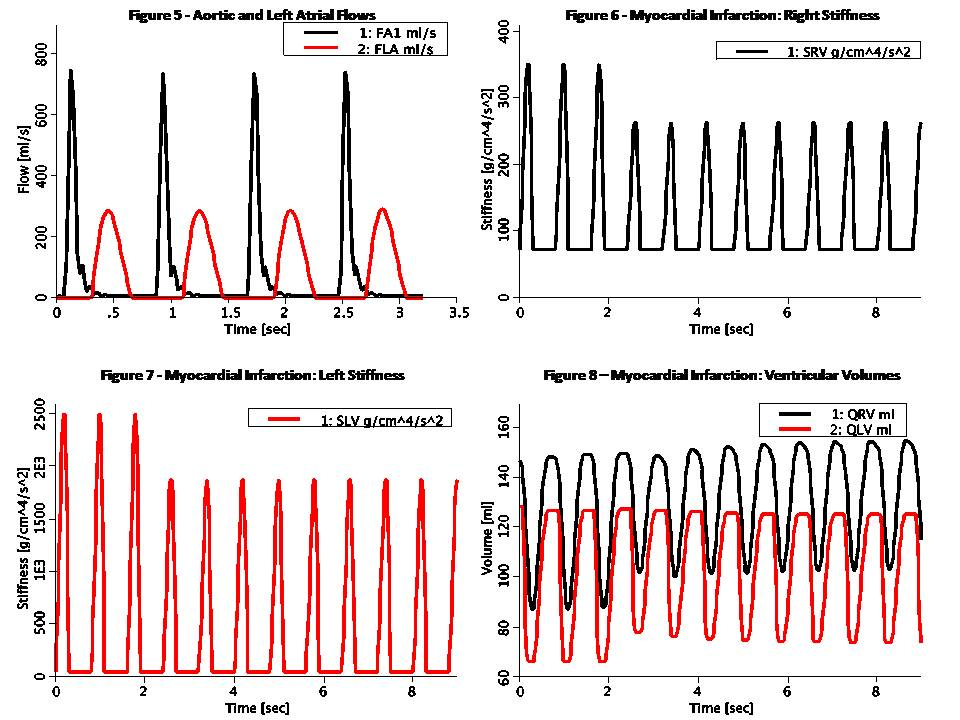# Rideout_PressureFlow1

Model number
0343

Uncontrolled CV loop pressure-flow model ported from Rideout (ACSL program PF-1). Also in MATLAB.

## Description```This model simulates the complete CV loop using Pressure-Flow modeling.
The model is uncontrolled, i.e. does not include baroreceptor sensor
connections to the central nervous system (CNS). Also, it does not
consider blood diffusion from and to tissue. The model takes advantage
of the analogy to RLC circuits as shown below:

Pressure-Flow-Volume             Electrical Circuit Equivalent
------------------------------------------------------------------
F  Flow (ml/s)                   I  Current (Amper)
P  Pressure (mmHg or g/cm/s^2)   V  Voltage (Volt)
Q  Volume (ml)                   Q  Charge (Coulomb or Amper*s)
R  Resistance (g/cm^4/s)         R  Resistance (Ohm or Volt/Amper)
C  Compliance (cm^4*s^2/g)       C  Capacitance (Farad or s/Ohm)
L  Inertance (g/cm^4)            L  Inductance (Henry or s*Ohm)

Pressure-Flow components obey the same fundamental equations as
their RLC circuit equivalents, i.e.:

P = R * F                        V = R * I    (Eq. 1 or Ohm's Law)
P = L * F:t                      V = L * I:t  (Eq. 2)
P = Q / C                        V = Q / C    (Eq. 3)
F = Q:t                          I = Q:t      (Eq. 4)

The venous segments of this model, both pulmonary and systemic, have a
structure much like the RLC circuit used in the artery in Rideout's left
heart model LH-PF-2, ported to JSim in model Rideout_PressureFlowLH. The
model has a single loop, but arteriovenous pathways may be easily added.

compliances are regarded as linear, but with unstressed volume (with
zero pressure). This is different than Rideout's CV loop model PF-0,
ported to JSim in model Rideout_PressureFlow0, which assumed no
unstressed volumes. In this model the inital condition in Eq. 4 above,
Q(0), consists of a stressed and unstressed components:

Q(0) = QU(0) + QS(0)

where QU = QU(0) is fixed. The pressure in Eq. 3 above, is obtained from
the stressed portion:

P = QS / C = (Q - QU) / C

Left and right ventricle stiffnesses SLV and SRV (S = 1 / C) in this
model use a periodic half-sine with a second harmonic added to shape the
actuator more realistically. Systole lasts 0.3 sec and diastole 0.5 sec,
such that the heart pulse is 0.8 sec (corresponding to 75 beats/min
heart pulse). Valves are simulated using flow limiters.

This model may be used to study the effect of myocardial infarction of
one or both ventricles introduced at a chosen time. It also allows study
of the effect of infusing or removing blood (bleeding) at the aorta at a
chosen time. These conditions are modeled with a JSim choice variable.

Figure 1 shows the ventricular volumes, QLV and QRV. Stroke volume
pumped out of each ventricle is 64 ml. At a heart pulse of 0.8 sec, this
corresponds to a output of 80 ml/sec (4.8 liter/min), which is
slightly low for an adult male.

Figure 2 shows the left ventricular locus on the Pressure-Volume plane.
Each systole-diastole cycle corresponds to one full counterclockwise
traversal of the loci. End-diastolic volume (EDV) is about 130 ml and
end-systolic volume (ESV) is around 66 ml. This means that the ejection
fraction (EDV - ESV) / EDV is about 50%.

Figure 3 shows the right ventricular and pulmonary artery pressures.
Figure 4 shows the left ventricular and aortic pressures. Figure 5 shows
the flow out of the first segments of the aorta (FA1) and left atrial
flow (mitral valve or left ventricular inflow). Oscillatory decay can be
observed after the aortic valve close.

The rest of the figures show the effect of a myocardial infarction. At
time 3.2 sec, systolic stiffness in both ventricles has been reduced by
25%, as seen in figures 6 and 7. Figure 8 shows the ventricular volumes.
Stroke volume is suddenly decreased at infarction time. A slow recovery
begins to occur as atrial average volumes and pressures increase. The
right ventricle end-diastolic volume, in particular, is seen increasing
and as stated by Starling's Law, stroke volume rises. This demonstrates
that the CV system is stable to a certain extent, even without CNS
control.
```

## Equations

The equations for this model may be viewed by running the JSim model applet and clicking on the Source tab at the bottom left of JSim's Run Time graphical user interface. The equations are written in JSim's Mathematical Modeling Language (MML). See the Introduction to MML and the MML Reference Manual. Additional documentation for MML can be found by using the search option at the Physiome home page.

References
```Rideout VC. Mathematical computer modeling of physiological systems.
Prentice Hall, Englewood Cliffs, NJ, 1991, Section 4.3, pp. 90-105
```
Key terms
Cardiovascular
CV
Aorta
Left
Right
Ventricle
Ventricular
Systemic Circulation
Pulmonary Circulation
Frank-Starling Mechanism
Uncontrolled
Myocardial Infarction
Blood Infusion
Bleeding
Heart Rate
Cardiac Output
Varying Elastance
Stiffness
Contraction
Muscle
Pressure-Flow-Volume
Ohm's Law
RLC circuit
Resistance
Resistive
Compliance
Compliant
Inertance
Stroke
Rideout
Acknowledgements

Please cite https://www.imagwiki.nibib.nih.gov/physiome in any publication for which this software is used and send one reprint to the address given below:
The National Simulation Resource, Director J. B. Bassingthwaighte, Department of Bioengineering, University of Washington, Seattle WA 98195-5061.

Model development and archiving support at https://www.imagwiki.nibib.nih.gov/physiome provided by the following grants: NIH U01HL122199 Analyzing the Cardiac Power Grid, 09/15/2015 - 05/31/2020, NIH/NIBIB BE08407 Software Integration, JSim and SBW 6/1/09-5/31/13; NIH/NHLBI T15 HL88516-01 Modeling for Heart, Lung and Blood: From Cell to Organ, 4/1/07-3/31/11; NSF BES-0506477 Adaptive Multi-Scale Model Simulation, 8/15/05-7/31/08; NIH/NHLBI R01 HL073598 Core 3: 3D Imaging and Computer Modeling of the Respiratory Tract, 9/1/04-8/31/09; as well as prior support from NIH/NCRR P41 RR01243 Simulation Resource in Circulatory Mass Transport and Exchange, 12/1/1980-11/30/01 and NIH/NIBIB R01 EB001973 JSim: A Simulation Analysis Platform, 3/1/02-2/28/07.